# 混沌Logistic映射的理论

## 混沌的基本概念

1975年，美国数学家约克和美籍华人李天岩发表了《周期3意味着混沌》的文章，首次提出了“混沌”—词，阐述了混沌的数学定义，对混沌学的发展具有重大意义。自此以后，混沌研究开始蓬勃发展。

## Logistic映射方程

Logistic映射是一个典型的非线性的迭代方程，如式所示：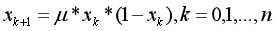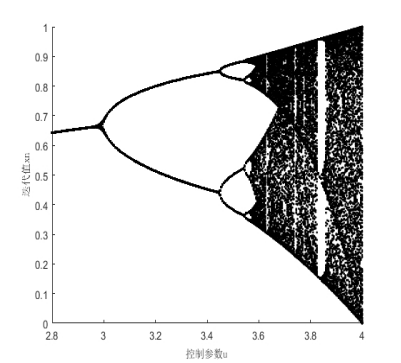# 混沌Logistic映射与其他加密算法介绍

## 普通行列置乱加密算法

### 普通置乱加密算法的流程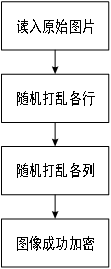### 算法分析

``````s = size(handles.img);
r = randsample(s(1), s(1));
RGBS = handles.img(r, :, :);
c = randsample(s(2), s(2));
RGBSS = RGBS(:, c, :);
axes(handles.axes2);
imshow(RGBSS);
title('普通置乱加密图像');
figure(2);
hist_im=histogram(RGBSS); %加密后直方图
title('普通置乱加密直方图');``````

## 像素点的RGB值缩放加密

### 像素点的RGB值缩放加密算法的流程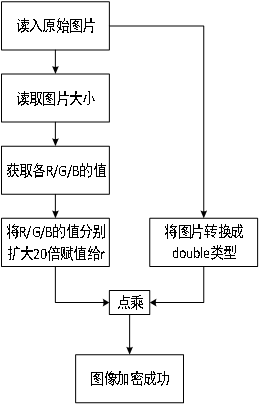### 算法分析

``````s = size(handles.img);
r = rand(s(1), s(2), s(3)) * 20;
RGBD = im2double(handles.img);
RGB_jiami = RGBD .* r;
axes(handles.axes2);
imshow(RGB_jiami);
title('像素点的RGB值缩放加密图像');
figure(3);
hist_im=histogram(RGB_jiami);
title('像素点的RGB值缩放加密直方图');``````

## 混沌Logistic映射加密算法

### 混沌Logistic映射加密算法模型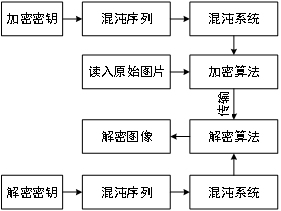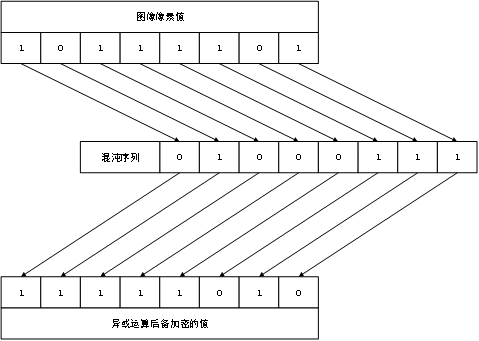### 算法分析

``````u=4;
for i=1:500
x=u*x*(1-x);
end
fprintf('x(k+1)=%d\n',x);
A=zeros(1,M*N);
A(1)=x;
for i=1:M*N-1
A(i+1)=u*A(i)*(1-A(i));
end
B=uint8(255*A); %
Imgn=reshape(B,M,N);
C=zeros(M,N);
for x=1:M
for y=1:N
C(x,y)=handles.img(x,y);
end
end
C;
D=uint8(C);
Rod=bitxor(D,Imgn);
Rod;
rod=reshape(Rod,M,N/3,3); ``````

# 验证与性能分析

## Matlab GUI操作界面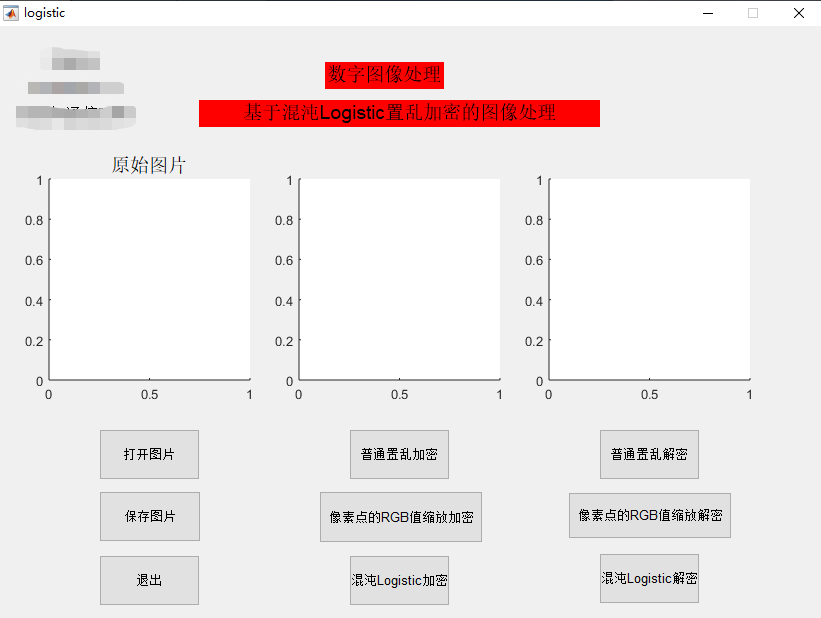## 普通行列置乱加密实现

Lena原图像、加密图像、解密图像Baboon原图像、加密图像、解密图像## 像素点的RGB值的缩放加密实现

Lena原图像、加密图像、解密图像Baboon原图像、加密图像、解密图像## 混沌Logistic映射加密实现

Lena原图像、加密图像、解密图像Baboon原图像、加密图像、解密图像## 直方图性能分析

Lena图的普通置乱与混沌Logistic加密的直方图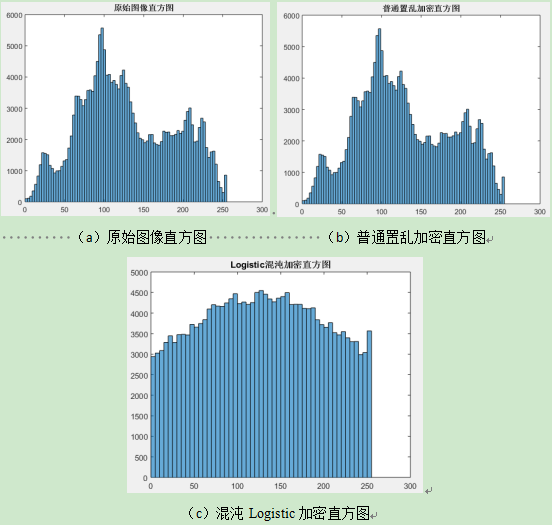Lena图的像素点的RGB缩放与混沌Logistic加密的直方图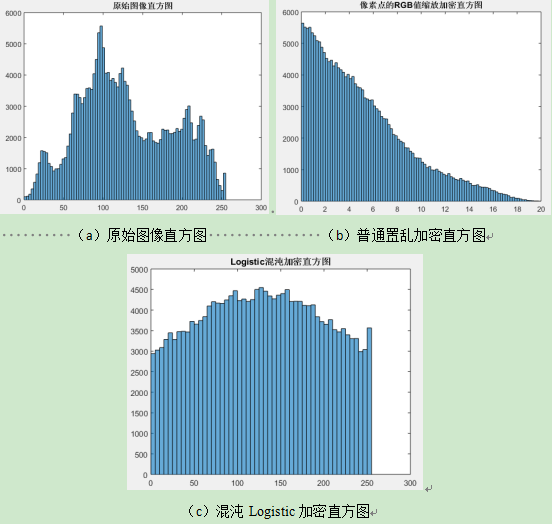转载规则

《基于混沌Logistic加密算法的图片加密与还原》 梦魇回生 采用 知识共享署名 4.0 国际许可协议 进行许可。
上一篇数学分析模型（一）：数据的无量纲处理方法及示例（附完整代码）

2020-12-01十大经典排序算法整理汇总（附代码）

2020-02-16
目录